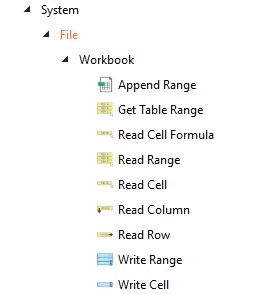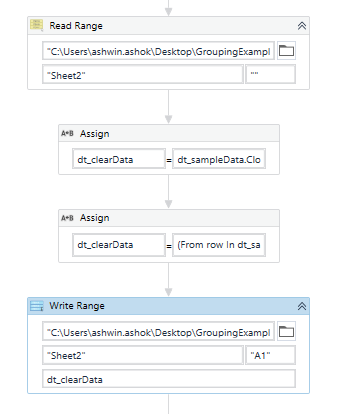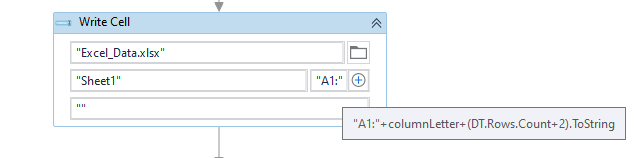# Delete Excel data range without Excel Installed

Is there a way to delete all the data from a sheet in excel without having excel installed on the machine bot will be running from?

I have a scenario where I need to read some data, manipulate, add, filter…then rewrite to the same sheet. Using only Write range will not work because if the beginning DT and the new DT are not the same size all the original data will not be overwritten…there will be leftovers.

So I need to read the original data then manipulate it using dt variables…then delete the original data from the sheet and rewrite the manipulated dt to the same excel sheet.

Any ideas how/if I can do this?

hey

for excel not installed you should use the workbook applicationsregards!

Hi @kasey.betts ,

If you only want to retain the headers, then we could try emptying the rows without deleting them before writing it back to Excel using the Workbook Write Range Activity``````(From row In dt_sampleData.AsEnumerable()
Let rw = row.ItemArray.ToList()
Let empty = rw.ConvertAll(Function(c) c.ToString.Replace(c.ToString,"")).ToArray()
``````

ClearDataFromExcelSheet.xaml (6.3 KB)

Kind Regards,
Ashwin A.K

@kasey.betts Why don’t you write the updated data to a different file with same name

• Read the data from actual file and store the file name in a variable. Move this file to a temporary folder
• Do manipulations in the data table
• Create different file in the same folder location where you got actual file, with same name as like the actual one and write the updated data using workbook write range

@kasey.betts , Assuming that we have the Data already available in Datatable, which Represents the Data in the Excel, we can use it’s Column Count and Row Count information to Clear the Contents using Write Cell Activity.

For Example :

1. Let the Datatable be `DT`

2. We get the Column Letter of the Excel by using the Column Count information as follows :

``````columnLetter = UiPath.Excel.Helpers.ExcelUtilities.ConvertColumnIndexToColumnLetter(DT.Columns.Count+1)
``````

The above would help us get the Column Letter in Excel.

1. Now using the Workbook Write Cell Activity, we can write Empty String into the Range below :
``````"A1:"+columnLetter+(DT.Rows.Count+2).ToString
``````We can Start from A2 or A1 depending on the need.

Try this and Let us know if it doesn’t work

1 Like

This topic was automatically closed 3 days after the last reply. New replies are no longer allowed.